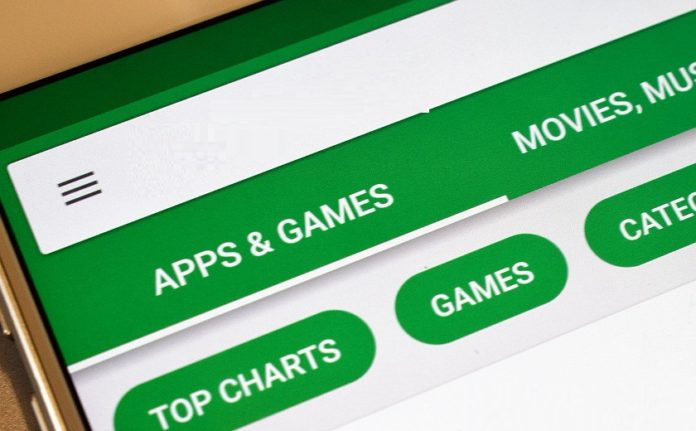We take a look on the best mathematical apps or best math apps for all kinds of equations, integrals, derivatives and more.  There are no limits and your mobile will thank you completely.

Today, Mathematics is a problem for many. They become essential in learning in adult life. We can already have many tools and apps for mathematical problem help.

We do not refer a math calculator apps.But we are refering the mathematical  apps and tools which will help you to pass any exam or test as well as help in solving the complex math problems or questions. You can also understand all kinds of concepts of maths so you can solve the most complex problems.

## HERE’RE THE BEST MATH APPS FOR IPHONE AND ANDROID MOBLIE USERS

### 1. GeoGebra

GeoGebra is a calculator app. GeoGebra is a scientific and mathematical app quite beneficial for all kinds of levels. GeoGebra has a list of really interesting features:

1. Draw the graphics.
2. You will have access to the computerized algebra next to the symbols.
3. Plotting of graphs.
4. Resolution of equations.
5. Interactive geometry
6. Calculations in sheets.
7. Have the resolution of every equation.
8. Search interactive geometry.
9. Have the calculation in the sheets.
10. Computerized algebra and problem solving with symbols.
11. Distributions and parameters in probability.

### 2. PCalc Lite

PCalc Lite is one of the best math apps around the world. PCalc Lite app is well deserve app for the ios user. PCalc Lite app is most powerful calculator app for the engineers, students, programmers, scienstist and other users.  PCalc Lite app have  lot of rich features of mathmatics which help to solving the complex problems of mathmatics. One more thing for users, they can also use this app in Apple Watch.

Whar is new in PCalc Lite ios app
1. PCalc Lite improve support for the new iPads users
2. Fixed the layouts importing problem or settings stored  problems on an iCloud Drive.
3. Fixed  the number displayed problems in the Settings app.

### 3. Smartick

Smartick is a best  math apps for the little childrens (primary level or under age10 ). Smartick app offers learning in a very intuitive and fun way. Smartick app will be helping everyone to better understand children’s mathematics.

Thousand of persons are using Smartick app for improving the children’s mathematics skills.

Smartick android app also offers all kinds of mathematical games and any exercise that will improve your child ability to solve any problem with in 15 minute in a day.

### 4. Mathematical formulas

Many people think that the math formulas apps are incredibly good  for mathematics. Most of people are using the several apps  for solving the math proplems. Mathematical formulas  app has all kinds of mathematical formulas for the field, be it geometry, algebra, arithmetic, analysis, logic or trigonometry and more.

### 5. Photo Math

In case you are looking for a tool to solve all types of mathematical operations, (They are also known as Math Solver App for their translation into English). I’ll tell you, it’s one of the best tools you can get for mathematical solutions. Imagine taking a picture of that problem that you have in your notebook and that the application solves it, because I will tell you, Photo Math works in that way.

It is a calculating tool with camera that has more success in this area. This is because there are no errors, you work well on derivatives, differential equations, integrals or any mathematical problem in general. In case you do not believe me, think it over and download it, check it yourself!

Also, not only solve any math problem from books, but has an intelligent software to recognize handwriting, this is ideal for any exam or task you have.

Work in general, for any branch of mathematics that you have thought, so, you should get all the benefits possible.